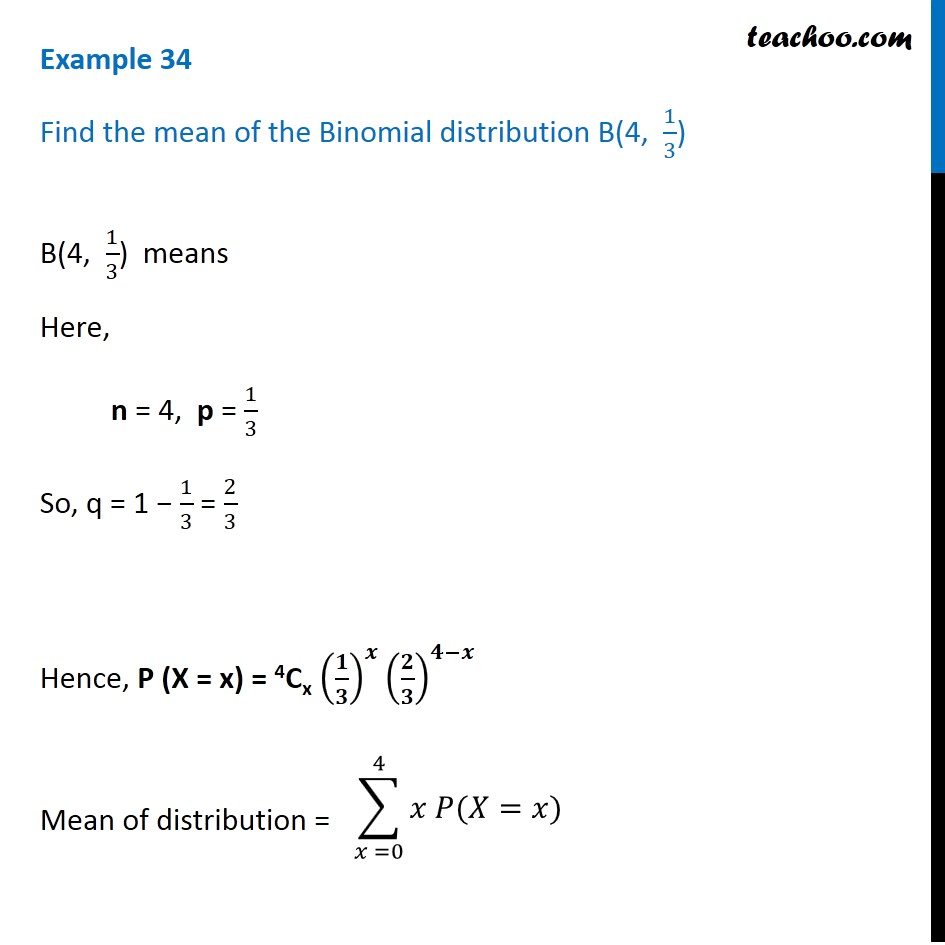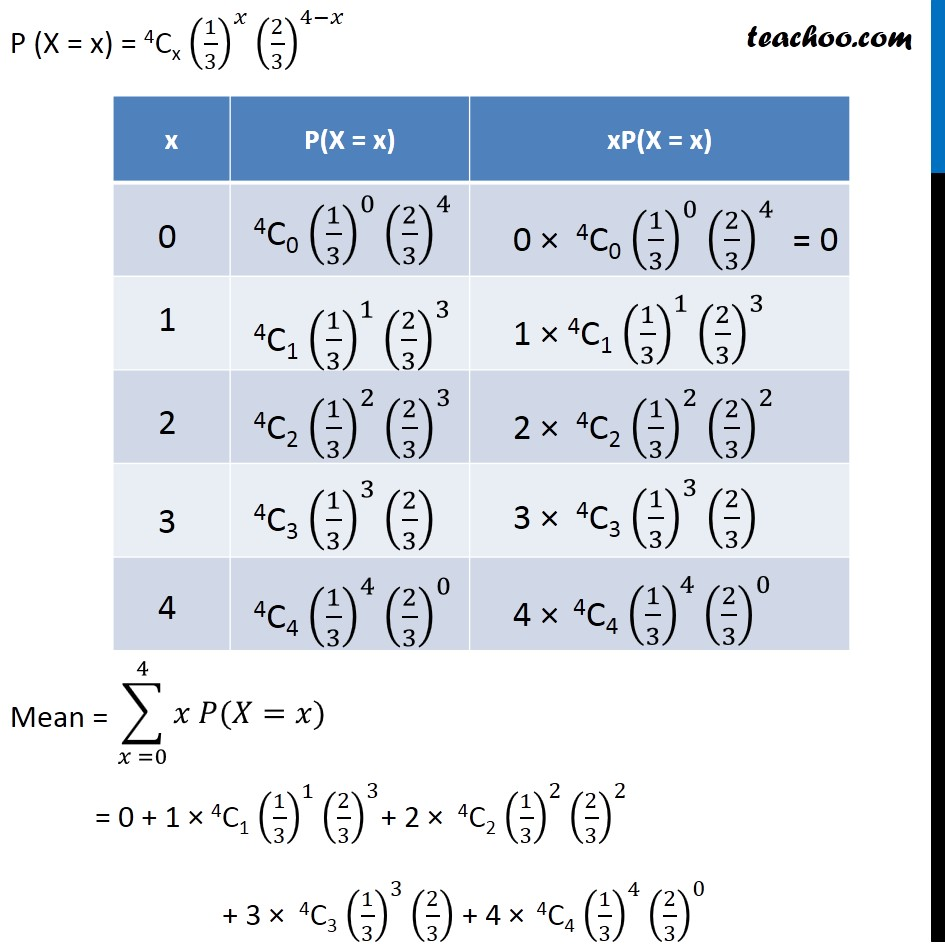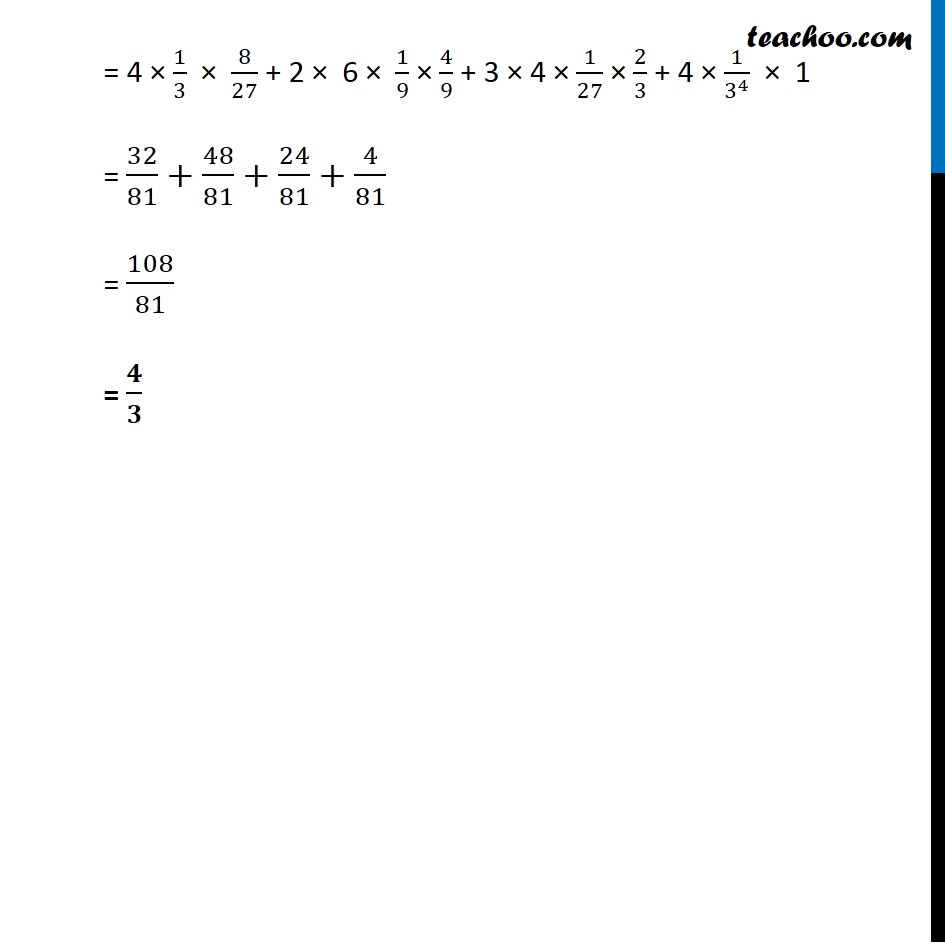Examples

Chapter 13 Class 12 Probability
Serial order wiseLearn in your speed, with individual attention - Teachoo Maths 1-on-1 Class

### Transcript

Question 12 Find the mean of the Binomial distribution B(4, 1/3) B(4, 1/3) means Here, n = 4, p = 1/3 So, q = 1 − 1/3 = 2/3 Hence, P (X = x) = 4Cx (𝟏/𝟑)^𝒙 (𝟐/𝟑)^(𝟒−𝒙) Mean of distribution = P (X = x) = 4Cx (1/3)^𝑥 (2/3)^(4−𝑥) Mean = = 0 + 1 × 4C1 (1/3)^1 (2/3)^3+ 2 × 4C2 (1/3)^2 (2/3)^2 + 3 × 4C3 (1/3)^3 (2/3) + 4 × 4C4 (1/3)^4 (2/3)^0 = 4 × 1/3 × 8/27 + 2 × 6 × 1/9 × 4/9 + 3 × 4 × 1/27 × 2/3 + 4 × 1/3^4 × 1 = 32/81+48/81+24/81+4/81 = 108/81 = 𝟒/𝟑